## Morphing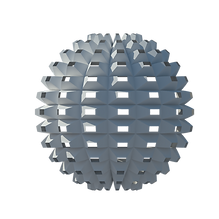## Modeling Process|     1. Modeling the basic module of the geometry     2.Combination of 2 basic module by using transformation techniques and generation of the developed module      3.Combination of the developed module and formation of the main geometry as a tower. GC Tool: Generated Node Technique (GNT) Transformation and Replication Concepts by using CoordinateSystem.ByUniversalTransform Double Skin Tower Modeling | First Skin Level of Zooming: Geometry Subdivision Parametric Design Concepts: Place Holder      1. by using the GNT providing one Module of Window and window frame (Place Holder)     2. Point grid on one side of the building ( Replication, Jig) and Assigning the window to the point grid     3. Extension to all sides of the building ( Replication) Note: Final geometry assigned to all sides prevents large amount of calculation during the modeling and makes the file lighter. Double Skin Tower Modeling | Second Skin Level of Zooming: Geometry Subdivision Parametric Design Concepts: Point Collection, Rationalization Note1: Using GNT( Generated Node Technique) in most of the parts Modeling Process|     1. Generation of Random points on one side of the cube         (Delaunay diagram)     2. Delaunay triangulation     3.Extension to all sides of the building ( Replication) Note 2: Final geometry assigned to all sides prevents large amount of calculation during the modeling and makes the file lighter.## PARAMETRIC MODELING

These examples are done in

"Parametric Modeling" Course, And Grasshopper Environment

Special Thanks to Professor Ramesh Krishnamurti

Fall 2012

Spiral Curve along a Circle

By using the Function and the Point Polar component a spiral along a given 3D curve can be generated. The grasshopper file needs to have at least two numeric controllers for number of segments and total number of rotations. Also, the sampling points from the given curve for constructing the spiral should be evenly distributed. By adding another set of parameters this curve can be modified.

The concept of this modeling is related to landscape furniture design.Rose Curves Modeling

By using the equation for the rose curve and the Function component with three input variables (x,y,z) a series of 2D and 3D Rose curves can be derived.

The following equation is used for constructing points on the rose curve and is based on polar coordinate system, where a point is defined by 𝑃(𝛾, 𝜃).

Equation for Rose Curve Point :        𝛾 = sin (𝜅𝜃)   (𝜅 =𝑛 /𝑑)

Based on Rose Curve modeling, a turing tower can be modeled in grasshopper. And in accordance with different amounts for n and d , different forms for tower can be generated.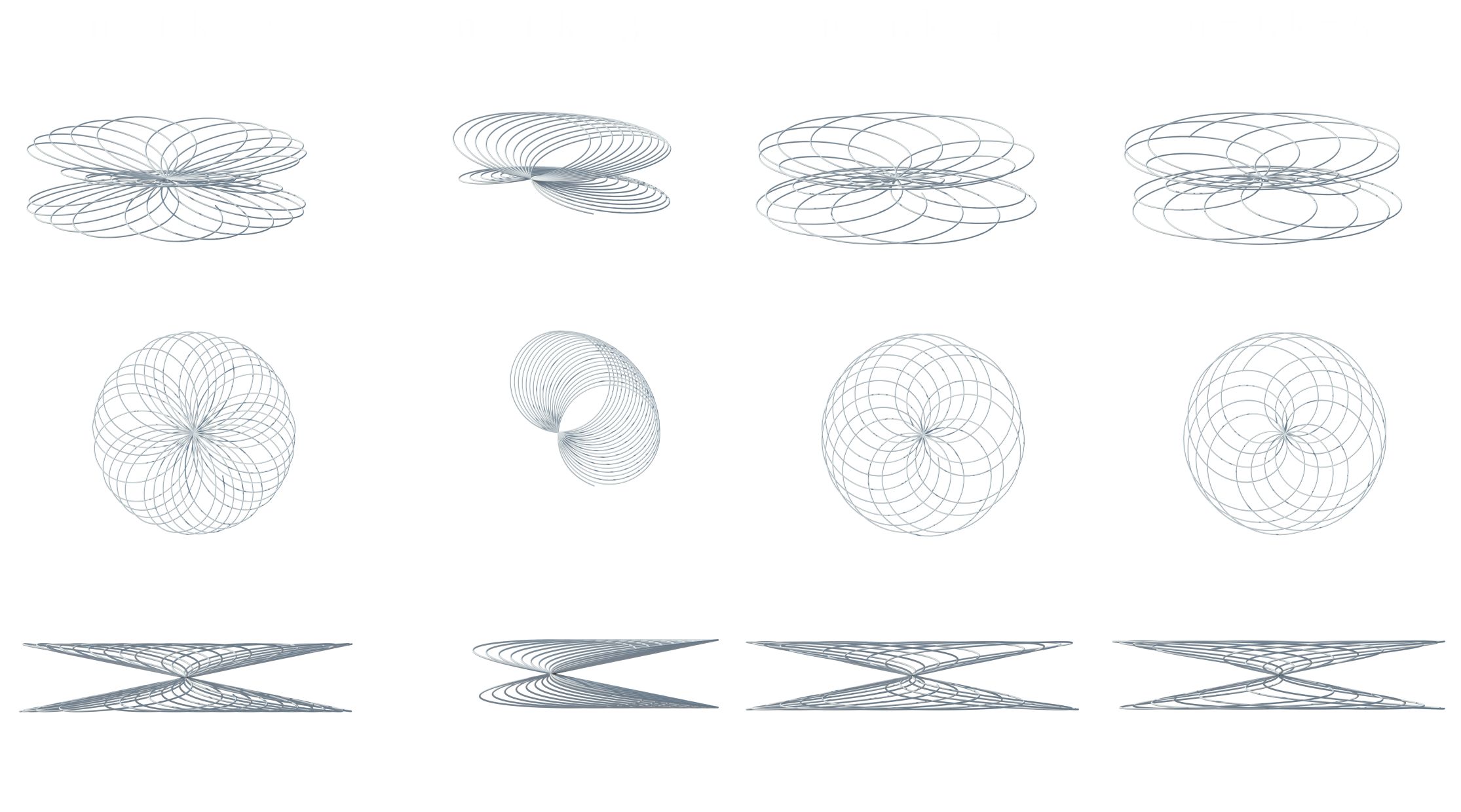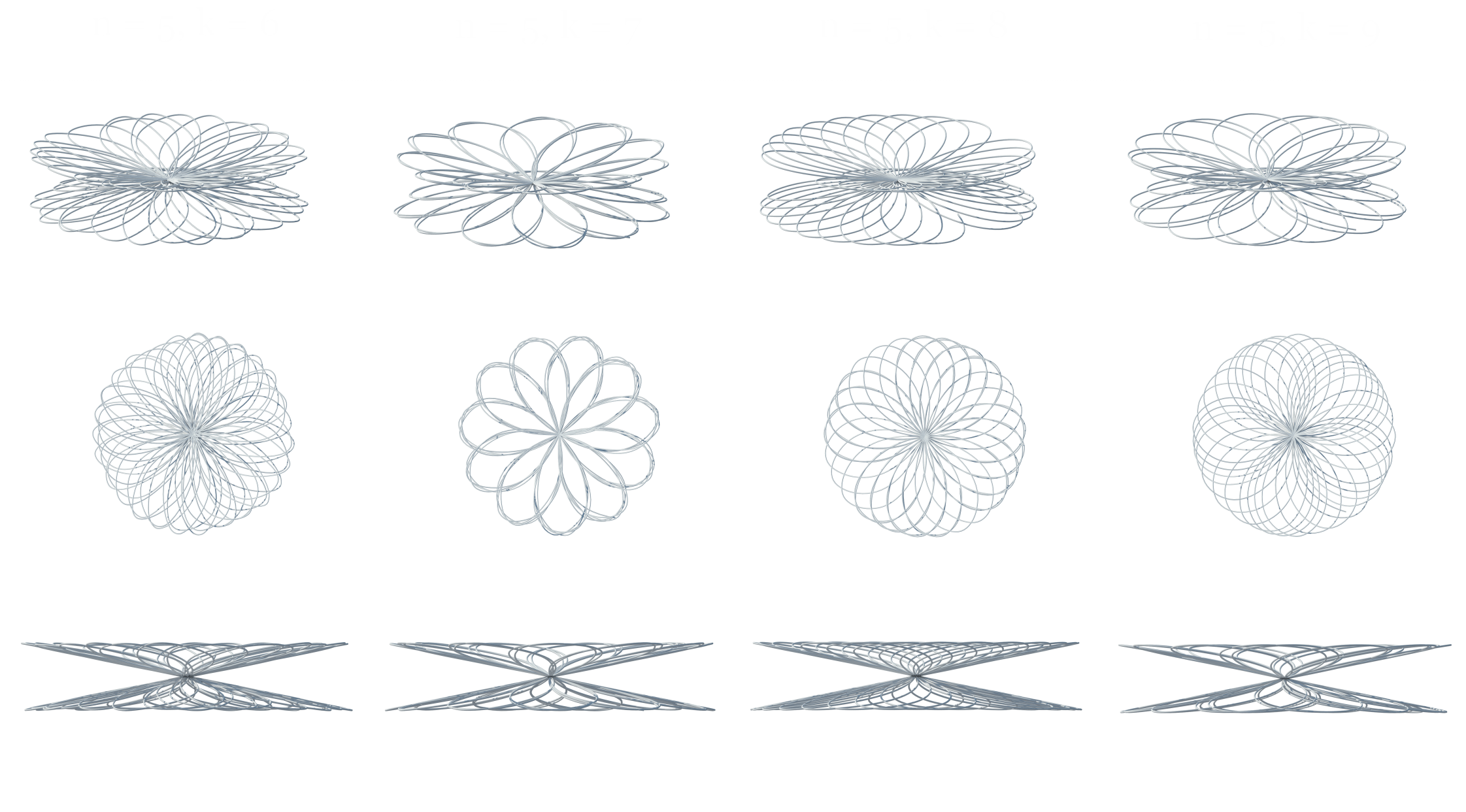Remapping HexGrid Surface

By starting from a basic HexGrid component in grasshopper, a hexagonal pattern on any given surface will be created. We should construct the parametric relationship for the hexagonal pattern by examining the HexGrid component. We could then reuse this relationship to generated the hexagonal grid on any untrimmed surface. The relationship should be parametric both ways. If the original hexagonal pattern be changed, then the mapped pattern on the surface should be updated accordingly. If the surface be deformed, then the mapping of the hexagonal pattern should be adjusted. This parametric relationship expresses how a hexagonal pattern can be generated on a surface by specifying the underlying UV parameters.Experimenting with Mobious Strip

A Mobius strip is an example of moving a line segment ‘g’ along a circle ’ c’.  As moving the line segment ‘g’ along ‘c’, ‘g’ is continuously rotated around ‘c’ and remain orthogonal to the same intersecting point . When ‘g’ returns to the starting position it should turn the rotation angle,   u = pSelf - Intersecting Mobius Strip

For every point on this Mobius Strip surface:

X = sin(2*u)*(-­‐2+v*sin(u/2))

Y = cos(2*u)*(-­‐2+v*sin(u/2))

Z = v*cos(u/2)where0

(0<u<2π, ­‐1<v<1)

Based on simple Mobius strip definition, Function components with Surface from Points component are combined to generate the surface. In next step an unrolled strip is created, which has the same length of the Mobius strip. The radius for these trimmed openings, circles, has a ratio to the length of this strip:

r = (length / numofOpenings) / c

where c is the control factor that changes the size of the openings## Parametric Pavilion

The objective of this exercise is to generate a parametric pavilion in order to experiment with surface tessellations.## Mesh Refinement via Edge Midpoint Insertion

Instead of using the mesh component directly, 4Point Surface component is used for this exercise. Each 4Point Surface is treated as a quadrilateral mesh, which consists of 4 vertices {A, B, C, D}. Given any free‐form surface from Rhino (or initiated in Grasshopper), the first step is to decompose the surface into a number of sub- surfaces.

The second step is then to insert edge midpoints of each face and connect  these new points to create the central quads. The final step is to fill the gaps between these new quads. The final refinement output yields diamond‐like (diagrid) configuration.

3D Diamond Box Morphing

Twisted Box component rather than surface boxes in this problem for box morphing has been used. The Twisted Box is a component that allows you to create a reference (or target) box from scratch by Specifying eight vertices of the box.  In this problem, you are asked to create the diamond boxes and use them for the surface tessellation with potential panel modules.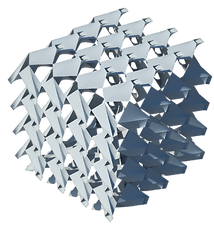A responsive surface that will respond to the input stimulus is created. For example, the sun position can be treated as the input parameter. Here, a parametric façade is designed which consists of number of surface components and they will respond to the change of the attracting resources respectively.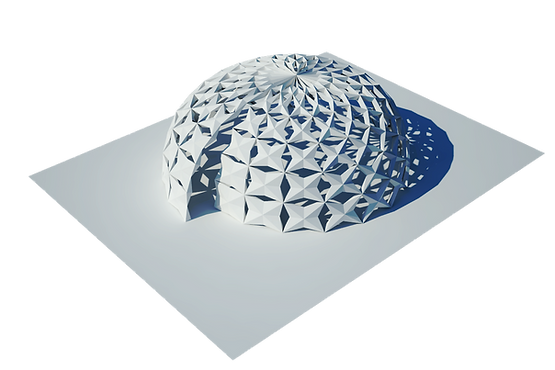Surface Subdivision by Single / Multiple attractors

We can modify a two dimensional domain, defined by {{u0,u1},{v0,v1}}, to generate the customized grid pattern for surface subdivision. By utilizing the Graph component to vary the density of the grid pattern successfully.

Here, attractors are introduced as references to drive the surface subdivision pattern.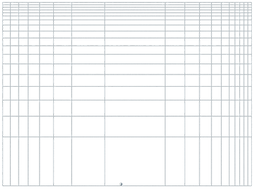VORONOI TESSELATION

, a Voronoi diagram is a way of dividing space into a number of regions. A set of points (called seeds, sites, or generators) is specified beforehand and for each seed there will be a corresponding region consisting of all points closer to that seed than to any other. The regions are called Voronoi cells.(wikipedia)private void RunScript(List<Point3d> pointSet, List<Point3d> boundary, ref object polyLines, ref object curves)
{

//CHECK EDGE CONDITIONS
if(pointSet.Count < 1 || boundary.Count < 1){
Print("Input PointSet, or Boundary Points, is null");
return;
}

//INITIALIZE LISTS TO STORE OUTPUT CURVES AND OUTPUT POLYLINES
List<Curve> vCurves = new List<Curve>();
List<Polyline> vPLines = new List<Polyline>();

List<VoronoiCell> vCells = new List<VoronoiCell>();

//ITERATE OVER EACH POINT IN THE INPUT POINTSET
foreach(Point3d p in pointSet) {

//instantiate a voronoi cell for each point
VoronoiCell vCell = new VoronoiCell(p, boundary);

foreach(Point3d pt in pointSet) {

//we need to consider all points BUT the point under consideration
if (pt != p) {
vCell.sliceCell(pt);
}
}
}

Print("\nNumber of polyLines: " + vPLines.Count);
Print("Number of OnNurbsCurve: " + vCurves.Count);

//SET THE OUTPUTS
polyLines = vPLines;
curves = vCurves;

}

public class VoronoiCell {
//VARIABLES
List<Point3d> vertices;
Point3d center;

//CONSTRUCTOR
public VoronoiCell(Point3d p, List<Point3d> pts) {
this.center = p;
this.vertices = pts;
}

//METHODS

// Slice Cell operation
public void sliceCell(Point3d pt) {
// list for storing temporary new boundary points
List<Point3d> tempVertices = new List<Point3d>();
// list for storing final new boundary points
List<Point3d> newVertices = new List<Point3d>();

// counter for number of intersection of bisector with current boundary
int numIntersections = 0;
// intersection of a boundary segment with bisector
double intersection;

//STEP 1: CREATE BISECTOR
Line segment01 = createBisector(this.center, pt);

//STEP 2: FIND INTERSECTING POINTS
for (int i = 0; i < this.vertices.Count; i++) {

// create a boundary segment by connecting two sequential points in vertices array
Line segment02 = new Line(this.vertices[i], this.vertices[(i + 1) % vertices.Count]);

// add first point of segment to new boundary

// if a valid intersection found by current boundary segment
// add the intersection point as a new point to boundary points list

intersection = intersect2Lines(segment02, segment01);
if (intersection != -1) {

// a new intersection has been found
numIntersections++;
}

}

//STEP 3:UPDATE BOUNDARY VERTICES
if (numIntersections == 2) {

// two doubles could not be exactly equal
// so there is an epsillon tolerance for finding distances

double epsillon = 0.0001;

// traverse trough all points in new vertices and find points
// that belongs to this cell and remove others
// note: points that belongs to this cell is closer or have equal distance to
// cell center compare to point to slice from "pt"

foreach(Point3d newVerticesPt in tempVertices) {
if ((newVerticesPt.DistanceTo(this.center) - newVerticesPt.DistanceTo(pt)) < epsillon) {
}
}

// update current boundary by replacing it with
// new boundary

this.vertices = newVertices;
}
}

public Line createBisector(Point3d ptStart, Point3d ptEnd) {
Point3d newPtEnd = new Point3d();

Point3d newPtStart = (ptStart + ptEnd ) / 2;
newPtEnd.X = newPtStart.X - (ptEnd.Y - ptStart.Y);
newPtEnd.Y = newPtStart.Y + (ptEnd.X - ptStart.X);

return new Line(newPtStart, newPtEnd);
}

/*
*This method returns a double value representing the intersection
*of two lines. If the value is equal to -1 the lines do not
*intersect; otherwise the value represents the parameter to locate
*the intersecting point.
*/

public double intersect2Lines(Line line01, Line line02) {
double D; // Denominator
double E; // Enumerator

//line01
double line01startX = line01.From.X;
double line01endX = line01.To.X;
double line01startY = line01.From.Y;
double line01endY = line01.To.Y;

//line02
double line02startX = line02.From.X;
double line02endX = line02.To.X;
double line02startY = line02.From.Y;
double line02endY = line02.To.Y;

D = (line02endY - line02startY) * (line01endX - line01startX)
- (line01endY - line01startY) * (line02endX - line02startX);

if (D == 0) return -1.0;

E = (line02endX - line02startX) * (line01startY - line02startY)
- (line02endY - line02startY) * (line01startX - line02startX);

//intersection function - parameter to locate the intersecting point with the line range
double intersection = E / D;
if (intersection < 0 || intersection > 1) return -1.0;

return intersection;
}

/*
*This method returns the points maintained by the VoronoiCell stored in an Array. Also the first point of the initial list is also added at the end. This method is called by the createCrv()method in order to get the points for generating a closed curve,
*/

private Point3d[] get3DPoints() {
List<Point3d> points = new List<Point3d>();

//repeat the first point to the end of the list
return points.ToArray();
}

public Curve createCrv(int degree) {

NurbsCurve nCurve = NurbsCurve.Create(true, degree, this.vertices);

return nCurve;
}

public Polyline createPolyline() {

// create a closed polyline by connecting cell boundary points
Polyline boundaryPolyline = new Polyline();
for(int i = 0; i <= this.vertices.Count; i++) {
}
return boundaryPolyline;
}

}DELAUNAY TRIANGULATION

In mathematics and computational geometry, a Delaunay triangulation for a set P of points in a plane is a triangulation DT(P) such that no point in P is inside the circumcircle of any triangle in DT(P). Delaunay triangulations maximize the minimum angle of all the angles of the triangles in the triangulation; they tend to avoid skinny triangles.

For a set of points on the same line there is no Delaunay triangulation. For four or more points on the same circle (e.g., the vertices of a rectangle) the Delaunay triangulation is not unique: each of the two possible triangulations that split the quadrangle into two triangles satisfies the "Delaunay condition", i.e., the requirement that the circumcircles of all triangles have empty interiors.

By considering circumscribed spheres, the notion of Delaunay triangulation extends to three and higher dimensions. Generalizations are possible to metrics other than Euclidean. However in these cases a Delaunay triangulation is not guaranteed to exist or be unique.(Wikipedia)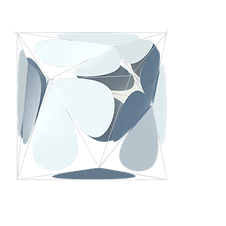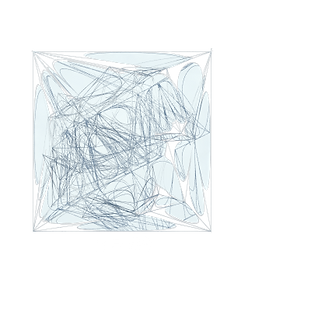private void RunScript(List<Point3d> pointSet, List<Point3d> boundary, ref object polyLines, ref object curve)
{
circumCircle c;
List<Point3d> triangles = new List<Point3d>();
for (int i = 0; i < pointSet.Count - 2;i++){
for (int j = i + 1; j < pointSet.Count - 1;j++){
for (int k = j + 1; k < pointSet.Count;k++){
c = new circumCircle(pointSet[i], pointSet[j], pointSet[k]);
bool pointValid = true;
for (int l = 0; l < pointSet.Count && pointValid;l++){
if (l != i && l != j && l != k)
{
if (c.IsPointInside(pointSet[l]))
{
pointValid = false;
}
}
}
if (pointValid)
{
}
}
}
}
List<Polyline> poly = new List<Polyline>();
List<Curve> vCurves = new List<Curve>();
Polyline boundaryPolyline = new Polyline();
List<Point3d> boundaryPoints = new List<Point3d>();
for(int i = 0; i < triangles.Count / 4; i++) {
boundaryPolyline = new Polyline();
boundaryPoints = new List<Point3d>();
for (int j = 0;j < 4;j++){
}
}
polyLines = poly;
curve = vCurves;
}

BOID FLOCKING

Boids is an artificial life program which simulates the flocking behavior of birds. As with most artificial life simulations, Boid is an example of emergent behavior; the complexity of Boids arises from the interaction of individual agents adhering to a set of simple rules.

These rules are:

separation: steer to avoid crowding local flockmates

alignment: steer towards the average heading of local flockmates

cohesion: steer to move toward the average position (center of mass) of local flockmates.

(wikipedia)Rhino Script : C# Coding in Grasshopper

private void RunScript(List<On3dPoint> P, List<On3dVector> D, double W, double L, double H, int S, ref object B_Loc, ref object B_Dir)
{
Print("Number of Flocking Boids:{0}\n\n", P.Count);
if(P.Count != D.Count) return;

// Add an initial set of boids into the system
if(flock == null)
{
Print("Flock is null");
flock = new Flock();
for (int i = 0; i < P.Count; i++)
{
flock.addBoid(new Boid(P[i], D[i], 50.0, 5, W, L, H));
}
}

flock.run();

B_Loc = flock.getBoidsLocs();
B_Dir = flock.getBoidsDirs();

}

Flock flock = null;// = new Flock();
static public Random ran = new Random();

public class Boid
{
public On3dPoint loc;
public On3dVector vel;
On3dVector acc;
double r;
double maxforce;    // Maximum steering force
double maxspeed;    // Maximum speed
double width;
double length;
double height;

public Boid(On3dPoint lc, On3dVector v, double mf, double ms, double wd, double len, double ht)
{
loc = lc;
acc = new On3dVector(0, 0, 0);
vel = v;
r = 2.0;
maxforce = mf;
maxspeed = ms;
width = wd;
length = len;
height = ht;
}

public void run(List<Boid> boids) {
flock(boids);
update();
borders();
}

// We accumulate a new acceleration each time based on three rules
void flock(List<Boid> boids)
{
On3dVector sep = separate(boids);   // Separation
On3dVector ali = align(boids);      // Alignment
On3dVector coh = cohesion(boids);   // Cohesion

// Arbitrarily weight these forces
//sep.mult(1.5); ali.mult(1.0); coh.mult(1.0);

sep *= (1.5);
ali *= (1 );
coh *= (1 );

// Add the force vectors to acceleration
acc += (sep);
acc += (ali);
acc += (coh);
}

// Method to update location
void update() {

// Update velocity
vel += (acc);

// Limit speed
if(vel.Length() > maxspeed)// Limit to maximum steering force
{
vel.Unitize();
vel *= maxspeed;
}

loc += (vel);

// Reset accelertion to 0 each cycle
acc *= (0);
}

// Wraparound
void borders() {
if (loc.x < -r) loc.x = width + r;
if (loc.y < -r) loc.y = length + r;
if( loc.z < -r) loc.z = height + r;
if (loc.x > width + r) loc.x = -r;
if (loc.y > length + r) loc.y = -r;
if( loc.z > height + r) loc.z = -r;
}

void render()
{

}

// Separation
// Method checks for nearby boids and steers away

public On3dVector separate(List<Boid> boids)
{
double desiredseparation = 10.0;//20.0;
On3dVector steer = new On3dVector(0, 0, 0);
int count = 0;

// For every boid in the system, check if it's too close
foreach( Boid b in boids)
{
double d = loc.DistanceTo(b.loc);
if((d > 0) && (d < desiredseparation))
{

// Calculate vector pointing away from neighbor
On3dVector diff = loc - b.loc;
diff.Unitize();
diff /= d; // Weight by distance

steer += diff;
count++;
}
}

if (count > 0) {
steer /= count;
}

// As long as the vector is greater than 0
if (steer.Length() > 0) {

// Implement Reynolds: Steering = Desired - Velocity
steer.Unitize();
steer *= maxspeed;
steer -= vel;

if(steer.Length() > maxforce)// Limit to maximum steering force
{
steer.Unitize();
steer *= maxforce;
}
}

return steer;
}

// Alignment
// For every nearby boid in the system, calculate the average velocity

public On3dVector align(List<Boid> boids)
{
double neighbordist = 15;//25.0;
On3dVector steer = new On3dVector(0, 0, 0);
int count = 0;
foreach( Boid b in boids)
{
double d = loc.DistanceTo(b.loc);

// If the distance is greater than 0 and less than an arbitrary amount (0 when you are yourself)
if((d > 0) && (d < neighbordist))
{
steer += b.vel;
count++;
}
}

if (count > 0) { // Average -- divide by how many
steer /= count;
}

// As long as the vector is greater than 0
if(steer.Length() > 0)
{
steer.Unitize();
steer *= maxspeed;
steer -= vel;

if(steer.Length() > maxforce)
{
steer.Unitize();
steer *= maxforce;
}
}
return steer;
}

// Cohesion
// For the average location (i.e. center) of all nearby boids,
// calculate steering vector towards that location

public On3dVector cohesion(List<Boid> boids)
{
double neighbordist = 5;//25.0;

On3dVector sum = new On3dVector(0, 0, 0);

int count = 0;
foreach(Boid b in boids)
{
double d = loc.DistanceTo(b.loc);
if((d > 0) && (d < neighbordist))
{
sum += b.loc;
count++;
}
}

if(count > 0)
{
sum /= count;
return steer(sum, false);
}

return sum;
}
public On3dVector steer(On3dVector target, bool slowdown) {
On3dVector steer;  // The steering vector
On3dVector desired = new On3dVector(target.x - loc.x, target.y - loc.y, target.z - loc.z);
double d = desired.Length();

// Distance from the target is the magnitude of the vector

// If the distance is greater than 0, calc steering (otherwise return zero vector)
if (d > 0)
{

// Normalize desired
desired.Unitize();

// Two options for desired vector magnitude (1 -- based on distance, 2 -- maxspeed)
if ((slowdown) && (d < 100.0)) desired *= (maxspeed * (d / 100.0)); // This damping is somewhat arbitrary
else desired *= (maxspeed);

// Steering = Desired minus Velocity
steer = new On3dVector(desired.x - vel.x, desired.y - vel.y, desired.z - vel.z);

if(steer.Length() > maxforce)// Limit to maximum steering force
{
steer.Unitize();
steer *= maxforce;
}
}
else
{
steer = new On3dVector(0, 0, 0);
}
return steer;
}

}

public class Flock
{
List<Boid> boids;

public Flock()
{
boids = new List<Boid>();
}

public void run()
{

// Passing the entire list of boids to each boid individually
foreach(Boid b in boids)
b.run(boids);
}

{
}

public List<On3dPoint> getBoidsLocs()
{
List<On3dPoint> locs = new List<On3dPoint>();

foreach(Boid b in boids)
{
}

return locs;
}

public List<On3dVector> getBoidsDirs()
{
List<On3dVector> dirs = new List<On3dVector>();
foreach(Boid b in boids)

return dirs;
}

}

RECURSIVE TRIANGULAR SUBDIVISION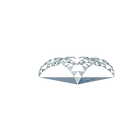Rhino Script : C# Coding in Grasshopper

private void RunScript(List<Point3d> pointList, int num, double H, ref object A, ref object B)
{

//CREATE A SURFACE OUT OF THE THREE POINT
Brep originalSurf = Brep.CreateFromCornerPoints(pointList, pointList, pointList, doc.ModelAbsoluteTolerance);

//MAKE A LIST AND ADD THE ORIGINAL SURFACE TO IT
List<Brep> surfaceList = new List<Brep>();

//GET THE MIDPOINTS OF THE EDGES OF THE ORIGINAL SURFACE
findEdgeMidPoints(originalSurf);

//RECURSIVELY SUBDIVIDE THE ORIGINAL SURFACE
List<Brep> subSurfaces = divideTriangles(surfaceList, H, num);

//REFERENCE OUTPUT VARIABLES
A = originalSurf;
B = subSurfaces;
}

/*
* THIS METHOD RECURSIVELY SUBDIVIDES AN INPUT TRIANGULAR SURFACE
* BY FINDING THE MIDPOINTS OF THE EDGES
*/

public List<Brep> divideTriangles(List<Brep> subSrf, double height, int N) {
//DEFINE A STOPPING CONDITION
if (N == 0) {
return subSrf;
}

//RECURSIVE PART
N--;

//make a list to add the new subsurfaces
List<Brep> newSubSrf = new List<Brep>();

//for every sub surface of the input list
foreach (Brep srf in subSrf) {

//call the method that gets the midpoints of the edges of the surface
//and fills triangles by connection the midpoints to the endpoints of
//the edges

List<Brep> tempSrfList = findEdgeMidPointsSubsurfaces(srf, height);
}
return divideTriangles(newSubSrf, height / 2, N);
}

public List<Point3d> findEdgeMidPoints(Brep surface) {
//1. GET THE EDGES FROM THE BREP
Curve[] edges = surface.DuplicateEdgeCurves();
List<Point3d> midPoints = new List<Point3d>();

//2. ITERATE OVER THE EDGES AND FIND MIDPOINTS
for(int i = 0; i < edges.Length; i++) {

//2a. GET THE EDGE
Curve thisEdge = edges[i];

//2B. GET THE MIDPOINT
Point3d midPoint = thisEdge.PointAt(thisEdge.GetLength() / 2);

//2C STORE IT IN THE LIST
}
return midPoints;
}

public List<Brep> findEdgeMidPointsSubsurfaces(Brep surface, double height) {

//1. GET THE EDGES FROM THE BREP
Curve[] edges = surface.DuplicateEdgeCurves();

//2. MAKE A LIST TO ADD THE NEW SUBSURFACES
List<Brep> subSurfaces = new  List<Brep>();

//3. GET THE NORMAL OF THE ORIGINAL FACE
Vector3d normal = surface.Faces.NormalAt(0, 0);

//4. ITERATE OVER THE EDGES AND FIND MIDPOINTS
for(int i = 0; i < edges.Length; i++) {

//GET THE ith EDGE
Curve thisEdge = edges[i];

//GET THE MIDPOINT OF THAT EDGE
Point3d thisMidPoint = thisEdge.PointAt(thisEdge.GetLength() / 2);

//MOVE THE POINT ALONG THE NORMAL VECTOR
thisMidPoint = thisMidPoint + normal * height;

//GET THE (i+1)th EDGE
Curve otherEdge = edges[(i + 1) % edges.Length];

//GET THE MIDPOINT OF THAT EDGE
Point3d otherMidPoint = otherEdge.PointAt(otherEdge.GetLength() / 2);

//MOVE THE POINT ALONG THE NORMAL VECTOR
otherMidPoint = otherMidPoint + normal * height;

//FIND THE ENDPOINT OF THE APPROPRIATE EDGE
Point3d endPoint = thisEdge.PointAtEnd;

//MAKE A SURFACE OUT OF THE TWO MIDPOINTS AND THE ENDPOINT OF THE iTH EDGE
Brep subSurf = Brep.CreateFromCornerPoints(thisMidPoint, endPoint, otherMidPoint, doc.ModelAbsoluteTolerance);

}
return subSurfaces;
}

SURFACE SUBDIVISION BY MULTIPLE ATTRACTORSRhino Script : C# Coding in Grasshopper

private void RunScript(Surface S, List<Point3d> Pts, int uNum, int vNum, ref object subSurface)
{

//Lists of x values and y values of attractors in seperate lists
List<double> Pts_x=new List<double>(),Pts_y = new List<double>();
foreach (Point3d pt in Pts) {
}

//Calculating the intervals between grids
double vInterval = S.Domain(1).Length / vNum;
double uInterval = S.Domain(0).Length / uNum;

//defining u and v in the grid
List<double> uList = new List<double>(),vList = new List<double>();
for (int i = 0;i < uNum ;i++){
}
for (int i = 0;i < vNum;i++){
}

//subSurf is the output of the script
List<Brep> subSurf = new List<Brep>();
Brep surf;

//finding domain values for both x and y
List<double> iso_u = new List<double>(),iso_v = new List<double>();
iso_u = isoCrvGen(Pts_x, uList);
iso_v = isoCrvGen(Pts_y, vList);

//Calculate the subsurface by having the domain values
Point3d p1,p2,p3,p4;
for (int i = 0;i < iso_u.Count - 1;i++){
for (int j = 0;j < iso_v.Count - 1;j++){
p1 = S.PointAt(iso_u[i] * uNum, iso_v[j] * vNum);
p2 = S.PointAt(iso_u[i + 1] * uNum, iso_v[j] * vNum);
p3 = S.PointAt(iso_u[i] * uNum, iso_v[j + 1] * vNum);
p4 = S.PointAt(iso_u[i + 1] * uNum, iso_v[j + 1] * vNum);

//creating each subsurface by the four points and adding it to the final subsurface list
surf = Brep.CreateFromCornerPoints(p1, p3, p4, p2, doc.ModelAbsoluteTolerance);
}
}

//OUTPUT
subSurface = subSurf;

}

//Here is a method which we do most of the calculations to get the domains
private List<double> isoCrvGen(List<double> Pts, List<double> list){
try{
foreach(double k in list){
double tempdist = 0;
for(int i = 0;i < Pts.Count;i++){

// Here we may have divide by zero so we add try catch to catch this exception
tempdist += 1 / Math.Abs((Pts[i] - k));
}
}
for(int i = 0;i < mathAdd.Count - 1;i++){
}